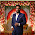# Checking if a number is Even or Odd using Bitwise Operator

Even Number: Any integer that is divisible by 2 is called even number. For example: 2,4,6,20 etc. are even numbers.

Odd Number: Any integer that is not divisible by 2, is called odd number. For example: 1, 3, 5, 7, 9 etc. are odd numbers.

In C/C++ or Java language, we can check whether a number is even or odd by using modulus operator. If an integer mod 2 is 0 then, it is even and if an integer mod 2 is 1 then, it is an odd number.

Even or Odd number can be tested by bitwise operator too. Bitwise & ( AND ) operator can be used to check for even or odd. If ANDing an integer by 1 results 1 then the integer is ODD else ( result 0 ) the integer is EVEN. But how does it work? Let's have a look at couple of examples. Let's take 9 as odd number and 14 as even number.

For 9:

```9 ->        1 0 0 1
1 ->     &  0 0 0 1
-------------------
result->    0 0 0 1
```

So 9 AND 1 gives us 1, as the right most bit of every odd number is 1.

For 14:

```14 ->      1 1 1 0
1  ->   &  0 0 0 1
------------------
result->   0 0 0 0
```

So 14 AND 1 gives us 0, as the right most bit of every even number is 0.

#### C Programming Implementation:

```#include <stdio.h>

int main()
{
int x;
printf("Enter a number: ");
scanf("%d", &x);
if(x&1)
printf("%d is ODD\n", x);
else
printf("%d is EVEN\n", x);
return 0;
}
```
Share:

#### 2 comments :

1.what does x&1 mean here?

2.Visit nice example Even odd logic

Spam comments will be deleted. :)